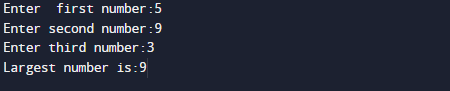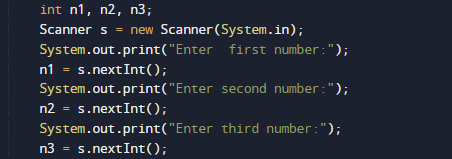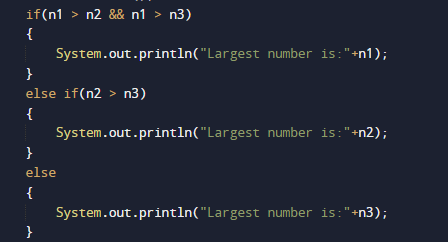# Java Program to find Largest of three numbers

In this tutorial you will learn about the Java Program to find Largest of three numbers and its application with practical example.

In this tutorial, we will learn to create Java Program to find Largest of three numbers using Java programming.

## Prerequisites

Before starting with this tutorial we assume that you are best aware of the following Java programming topics:

• Java Operators.
• Basic Input and Output function in Java.
• Class and Object in Java.
• Basic Java programming.
• If-else statements in Java.

## If statement

It consists a condition, followed by statement if condition true statement executed if not statement will not executed.

Syntax
if (Condition)
{
Statement;
}

## If-else statement

Test the condition. If condition true execute statement otherwise jump to else block and executed the statement.

Syntax:
if(condition)
{
code if condition is true;
}
else
{
code if condition is false;
}

### Java Program to find Largest of three numbers.

In this program we would find Largest of three numbers using Nested if-else statement . First of we would take three values from user and find the find smallest of three numbers . Let’s have a look at the code.

## OutputIn the above program, we have first declared and initialized a set variables required in the program.

• n1,n2 and n3 = it will hold entered numbers.

After that we take three numbers from user and find greatest among three using logical operator.After taking values from users we use logical operator and nested if else statement to calculate largest among three.

Then, we will find the greatest among  using if else statement.

• First of all we will check n1 is greater to both n2 and n3 ,then n1 is the greatest Else.
• Else n2 is greater n3 then n2 is the greatest.
• Else n3 is the greatest.And finally we print the result as shown in the image below.In this tutorial we have learn about the Java Program to find Largest of three numbers and its application with practical example. I hope you will like this tutorial.